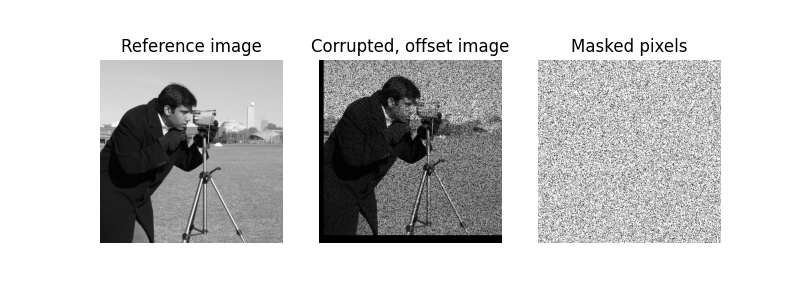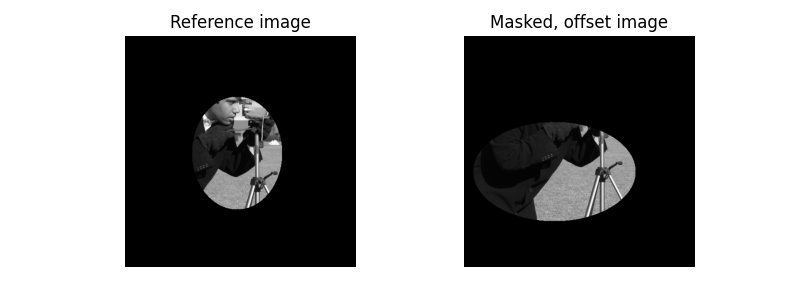In this example, we use the masked normalized cross-correlation to identify the relative shift between two similar images containing invalid data.

In this case, the images cannot simply be masked before computing the cross-correlation, as the masks will influence the computation. The influence of the masks must be removed from the cross-correlation, as is described in .

In this example, we register the translation between two images. However, one of the images has about 25% of the pixels which are corrupted.

```import numpy as np
import matplotlib.pyplot as plt

from skimage import data, draw
from skimage.registration import phase_cross_correlation
from scipy import ndimage as ndi
```

Define areas of the image which are invalid. Probability of an invalid pixel is 25%. This could be due to a faulty detector, or edges that are not affected by translation (e.g. moving object in a window). See reference paper for more examples

```image = data.camera()
shift = (-22, 13)

rng = np.random.default_rng()
corrupted_pixels = rng.choice([False, True], size=image.shape, p=[0.25, 0.75])

# The shift corresponds to the pixel offset relative to the reference image
offset_image = ndi.shift(image, shift)
offset_image *= corrupted_pixels
print(f'Known offset (row, col): {shift}')

# Determine what the mask is based on which pixels are invalid
# In this case, we know what the mask should be since we corrupted
# the pixels ourselves

detected_shift = phase_cross_correlation(image, offset_image,

print(f'Detected pixel offset (row, col): {-detected_shift}')

fig, (ax1, ax2, ax3) = plt.subplots(1, 3, sharex=True, sharey=True,
figsize=(8, 3))

ax1.imshow(image, cmap='gray')
ax1.set_axis_off()
ax1.set_title('Reference image')

ax2.imshow(offset_image.real, cmap='gray')
ax2.set_axis_off()
ax2.set_title('Corrupted, offset image')

ax3.set_axis_off()

plt.show()
``````Known offset (row, col): (-22, 13)

In scikit-image 0.21, phase_cross_correlation will start returning a tuple or 3 items (shift, error, phasediff) always. To enable the new return behavior and silence this warning, use return_error='always'.

Detected pixel offset (row, col): [-22.  13.]
```

Solid masks are another illustrating example. In this case, we have a limited view of an image and an offset image. The masks for these images need not be the same. The phase_cross_correlation function will correctly identify which part of the images should be compared.

```image = data.camera()
shift = (-22, 13)

shape=image.shape)

shape=image.shape)

offset_image = ndi.shift(image, shift)

print(f'Known offset (row, col): {shift}')

detected_shift = phase_cross_correlation(image, offset_image,

print(f'Detected pixel offset (row, col): {-detected_shift}')

fig = plt.figure(figsize=(8,3))
ax1 = plt.subplot(1, 2, 1)
ax2 = plt.subplot(1, 2, 2, sharex=ax1, sharey=ax1)

ax1.imshow(image, cmap='gray')
ax1.set_axis_off()
ax1.set_title('Reference image')

ax2.imshow(offset_image.real, cmap='gray')
ax2.set_axis_off()

plt.show()
``````Known offset (row, col): (-22, 13)